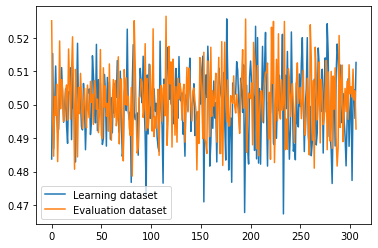# Help, i'm desperate: LSTM not learning anything no matter what parameters are used

So, i am trying to create an lstm for time series predictions one step further, problem is, it does not learn, at all, anything

I simplified my dataset to a simple sine wave, left my model with a bare-bone functionality: one lstm layer with hidden size 1000, and one 1000 to 1 linear layer with tanh activation after it, tried feeding to linear layer output[-1] and h_t outputs of lstm, played around with learning rate (from 0.0001 to 0.1), changed sequence length (from 11000 to 100), changed batch size (from 1 to 1024), but loss graph still looks like this:I’ve ran out of ideas on what is wrong, please, someone, help

Sine wave dataset:

``````class SineWaveDataset(Dataset):
def __init__(self,length, frequency, seq_length):
self.length = length
self.frequency = frequency
self.seq_length = seq_length + 1
def __len__(self):
return self.length // self.seq_length
def __getitem__(self,idx):
seq = np.array([np.sin(2*np.pi*self.frequency * (i/self.length)) for i in range(idx*self.seq_length,idx*self.seq_length+self.seq_length)])
#print("Seq length",len(seq))
feature = seq[0:len(seq)-1].astype('float32')
label = seq[-1].astype('float32')
return feature,label
``````

Model code:

``````class old_network(nn.Module):
def __init__(self, input_size=1, hidden_layer_size=100, output_size=1, seq_length_ = 1, batch_size_ = 128):
super().__init__()
self.hidden_layer_size = hidden_layer_size
self.batch_size = batch_size_
self.seq_length = seq_length_

self.lstm = nn.LSTM(input_size, hidden_layer_size, batch_first = False, num_layers = 1)

self.linear1 = nn.Linear(hidden_layer_size, output_size)
#self.linear2 = nn.Linear(hidden_layer_size // 2, output_size)
#self.relu1 = nn.ReLU()
self.tanh2 = nn.Tanh()

def forward(self, input_seq):
lstm_out, (h_out, _) = self.lstm(input_seq)
output = lstm_out[-1].reshape(self.batch_size,-1)
#print("Output shape ",output.shape)
#print("H_out shape: ",h_out.shape)
predictions = self.linear1(output)
#predictions = self.relu1(predictions)
#predictions = self.linear2(predictions)
predictions = self.tanh2(predictions)
#return predictions.permute(1,0,2)[-1]
return predictions
``````

Initialization code:

``````seq_length = 50
batch_size = 1024

model = old_network(input_size = 1, hidden_layer_size = 1000, output_size = 1, seq_length_ = seq_length, batch_size_ = batch_size).to(DEVICE)
loss_function = nn.MSELoss()

if os.path.exists(save_dir):
print("Models and optimizers loaded from checkpoint")

#dataset = h5FileDataset(data_dir, seq_length)
_length = 10000000
_frequency = 250000
dataset = SineWaveDataset(_length,_frequency, seq_length)
train_size = int(0.8*len(dataset))
test_size = len(dataset)-train_size
train_dataset, eval_dataset = random_split(dataset, [train_size,test_size])
batch_size=batch_size,
shuffle=True,
num_workers=0,
pin_memory=False,
drop_last=True)

batch_size=batch_size,
shuffle=True,
num_workers=0,
pin_memory=False,
drop_last=True)
``````

Learning loop:

``````losses = [[],[]]
epochs = 100
print("Starting training...")
try:
for epoch in range(epochs):
batch = 1
start = time.time()
seq = seq.reshape(seq_length,batch_size,1).to(DEVICE)
labels = labels.reshape(batch_size,1).to(DEVICE)

y_pred = model(seq)

loss = loss_function(y_pred, labels)
loss.backward()
optimizer.step()

try:
eval_seq, eval_labels = next(eval_iter)
except StopIteration:
eval_seq, eval_labels = next(eval_iter)
eval_seq = eval_seq.reshape(seq_length,batch_size,1).to(DEVICE)
eval_labels = eval_labels.reshape(batch_size,1).to(DEVICE)

eval_y_pred = model(eval_seq)

eval_loss = loss_function(eval_y_pred, eval_labels)
losses.append(eval_loss.item())
losses.append(loss.item())

print_inline("Epoch: {} Batch {}/{} Time/batch: {:.4f}, Loss: {:.4f} Loss_eval: {:.4f}".format(epoch,batch,batches,time.time()-start, loss.item(), eval_loss.item()))
batch += 1

if batch%25 == 0:
print("\n Epoch: {}/{} Batch:{} Loss_train:{:.4f} Loss_eval: {:.4f}".format(epoch,epochs,batch,loss.item(),eval_loss.item()))

plt.close()
plt.plot(range(0,len(losses)),losses, label = "Learning dataset")
plt.plot(range(0,len(losses)),losses, label = "Evaluation dataset")
plt.legend()
plt.show()
torch.save({'model_state_dict':model.state_dict(), 'optimizer_state_dict' : optimizer.state_dict()},save_dir)

except KeyboardInterrupt:
plt.close()
plt.plot(range(0,len(losses)),losses, label = "Learning dataset")
plt.plot(range(0,len(losses)),losses, label = "Evaluation dataset")
plt.legend()
plt.show()
``````